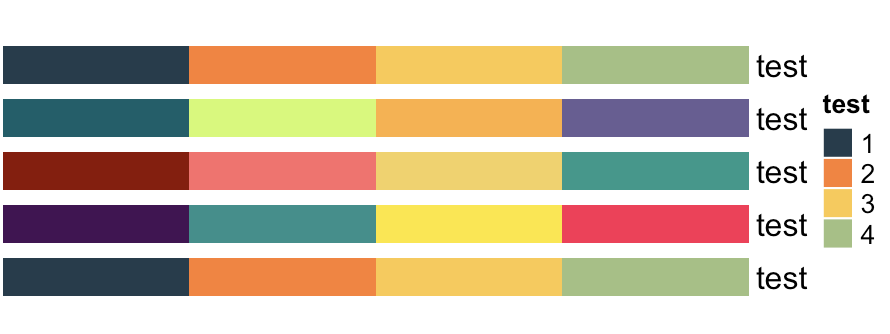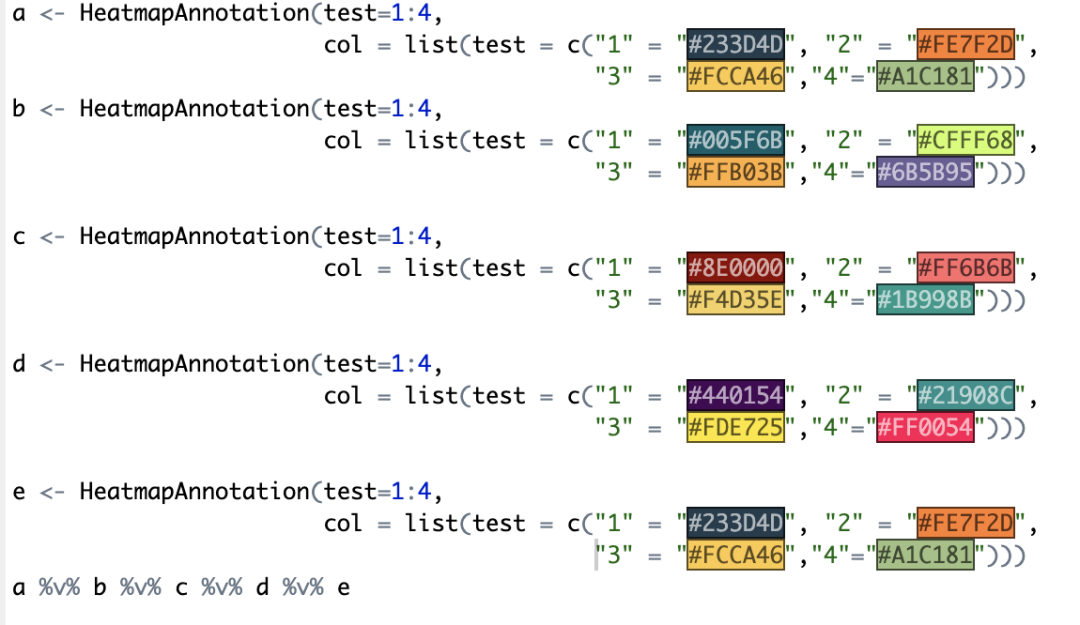# ChatGPT对科研绘图的帮助

chatgpt的回答：

a <- HeatmapAnnotation(test=1:4, col = list(test = c(“1” = “#233D4D”, “2” = “#FE7F2D”, “3” = “#FCCA46”,“4”=“#A1C181”)))b <- HeatmapAnnotation(test=1:4, col = list(test = c(“1” = “#005F6B”, “2” = “#CFFF68”, “3” = “#FFB03B”,“4”=“#6B5B95”)))c <- HeatmapAnnotation(test=1:4, col = list(test = c(“1” = “#8E0000”, “2” = “#FF6B6B”, “3” = “#F4D35E”,“4”=“#1B998B”)))d <- HeatmapAnnotation(test=1:4, col = list(test = c(“1” = “#440154”, “2” = “#21908C”, “3” = “#FDE725”,“4”=“#FF0054”)))e <- HeatmapAnnotation(test=1:4, col = list(test = c(“1” = “#233D4D”, “2” = “#FE7F2D”, “3” = “#FCCA46”,“4”=“#A1C181”)))a %v% b %v% c %v% d %v% e